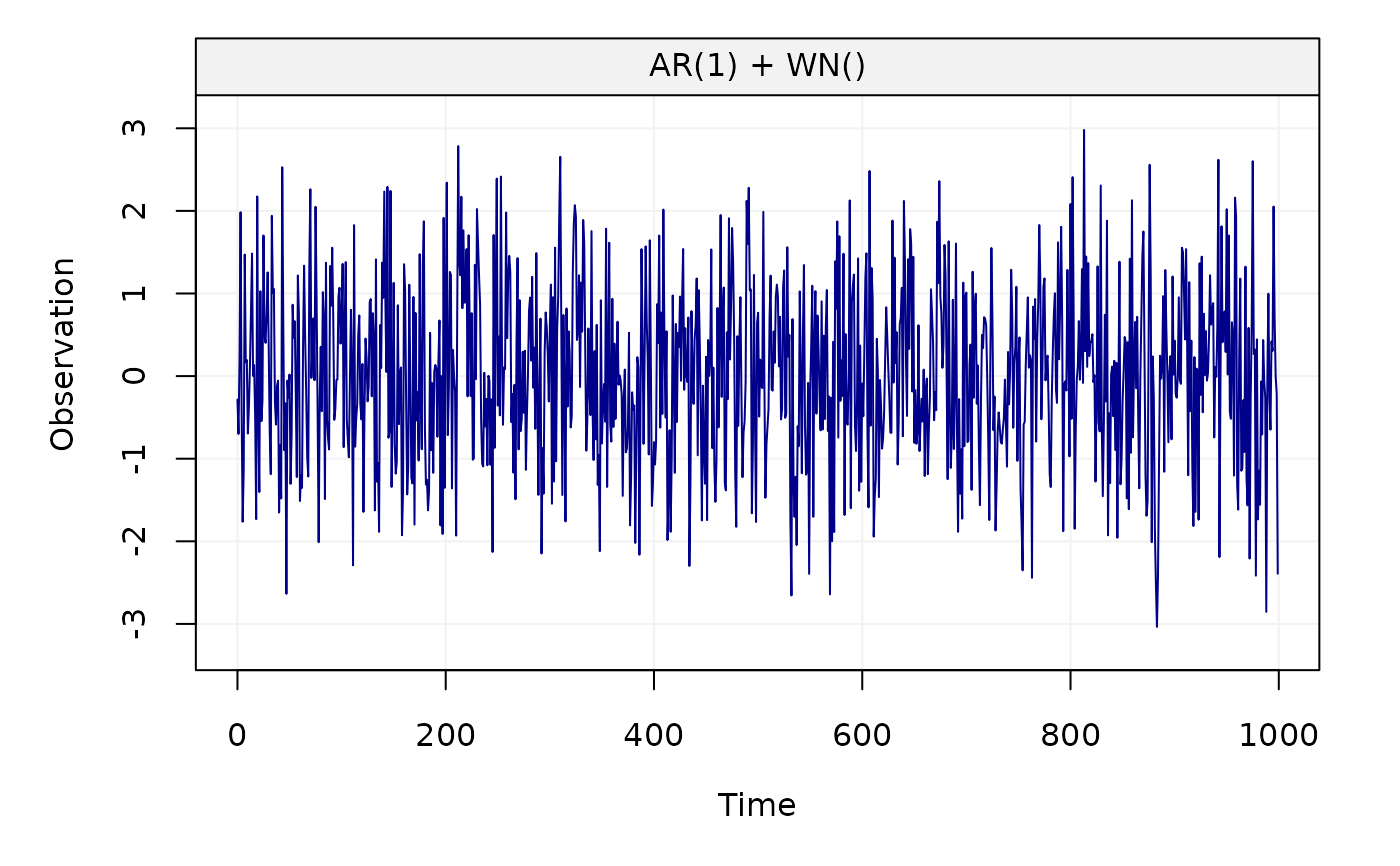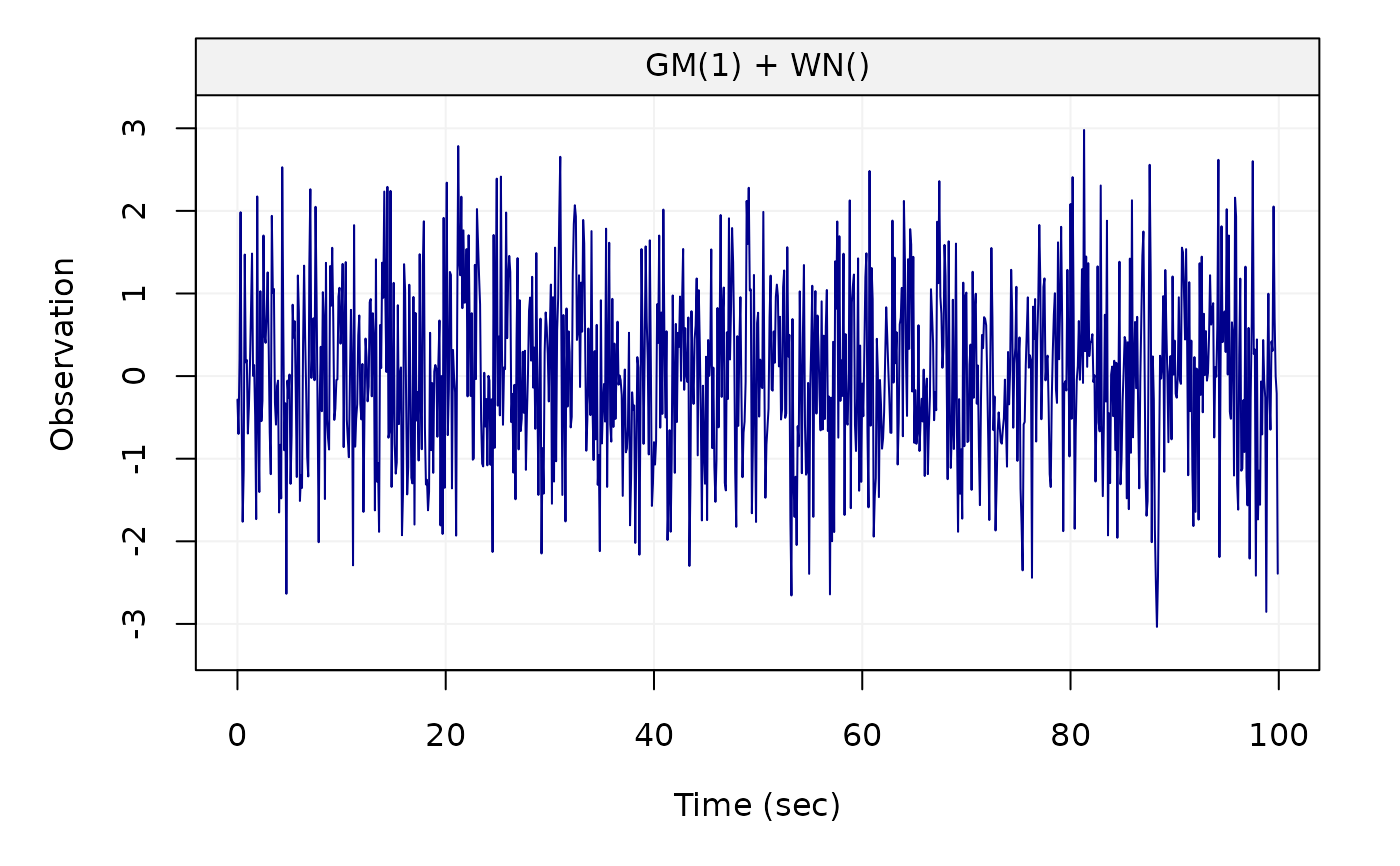Create a gts object based on a time series model.

gen_gts(
n,
model,
start = 0,
end = NULL,
freq = 1,
unit_ts = NULL,
unit_time = NULL,
name_ts = NULL,
name_time = NULL
)

## Arguments

n

An integer containing the length of the time series.

model

A ts.model or simts object containing the available models in the simts package.

start

A numeric that provides the time of the first observation.

end

A numeric that provides the time of the last observation.

freq

A numeric that provides the rate of samples. Default value is 1.

unit_ts

A string that contains the unit expression of the time series. Default value is NULL.

unit_time

A string that contains the unit expression of the time. Default value is NULL.

name_ts

A string that provides an identifier for the time series data. Default value is NULL.

name_time

A string that provides an identifier for the time. Default value is NULL.

## Value

A gts object

## Details

This function accepts either a ts.model object (e.g. AR1(phi = .3, sigma2 =1) + WN(sigma2 = 1)) or a simts object.

## Author

James Balamuta and Wenchao Yang

## Examples

# Set seed for reproducibility
set.seed(1336)
n = 1000

# AR1 + WN
model = AR1(phi = .5, sigma2 = .1) + WN(sigma2=1)
x = gen_gts(n, model)
plot(x)# Reset seed
set.seed(1336)

# GM + WN
# Convert from AR1 to GM values
m = ar1_to_gm(c(.5,.1),10)

# Beta = 6.9314718, Sigma2_gm = 0.1333333
model = GM(beta = m, sigma2_gm = m) + WN(sigma2=1)
x2 = gen_gts(n, model, freq = 10, unit_time = 'sec')
plot(x2)# Same time series
all.equal(x, x2, check.attributes = FALSE)
#>  TRUE